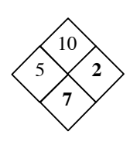### Home > AC > Chapter 1 > Lesson 1.2.1 > Problem1-44

1-44.

Copy and complete each of the Diamond Problems below. The pattern used in the Diamond Problems is shown below.1.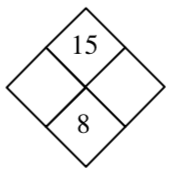1.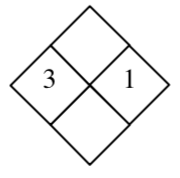1.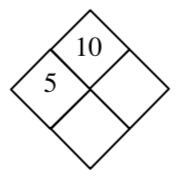1.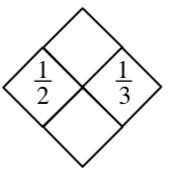1.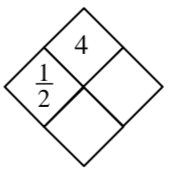1.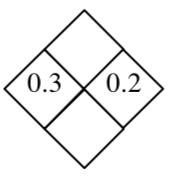1.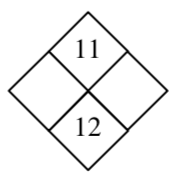1.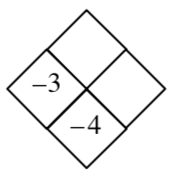Find out what values are missing from the diamond. (Is the $x$-value missing? $y$-value? $xy$ value? $x+y$ value?)

If the $x$ and $y$ value are missing, find what values can equal the top number, and what values equal the bottom number.

If the $xy$ value and the $x+y$ value are missing, use the values you do have (the $x$ value and the $y$ value) and multiply them together for the top value and add them together for the bottom value.

If the $x+y$ value and the $y$ value are missing: To find $y$: Find what number multiplied by $x$ equals the top number. To find $x+y$: Use the $y$ value you found and the $x$ value given and add them together.

If the $xy$ and $y$ value are missing: To find $y$: Use the $x$ value given and the $x+y$ value and subtract the $x$ value from the $x+y$ value. To find $xy$: Use the $y$ value you found and the $x$ value given and multiply.

1.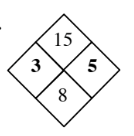1.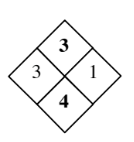1.